# IOS平台TensorFlow实践：逻辑斯蒂回归（附源码）（一）

+关注继续查看MATTHIJS HOLLEMANS

github地址：https://github.com/hollance# TensorFlow简介

TensorFlow是一个构建计算图（computational graphs）用来做机器学习的软件库。其他很多工具都以一种高抽象层次（higher level of abstraction）的方式工作着，比如通过Caffe，你能够设计一个不同层（layers）之间相互链接的神经网络（neural network）。 这和IOS上基础神经网络子程序(Basic Neural Network Subroutines, BNNS）和Metal 渲染（Metal Performance Shaders Convolution Neural Network，BPSCNN）提供的功能很相似。

# 使用逻辑斯蒂回归的二值分类

Note: 二值分类器虽然是最简单的分类器，但是其思想和那些能够区分成百上千类的分类器一样。虽然这篇文章中并没有进行深度学习，但某些理论基础是共同的。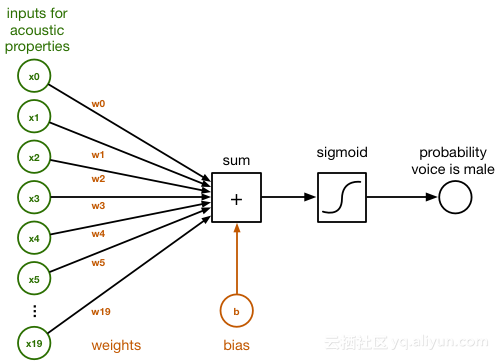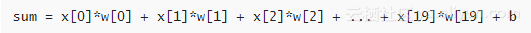训练分类器就是要找到w和b的正确的数值。初始化时，将w和b全部置0。训练多轮之后，分类器就会使用合适的w和b将男性声音和女性声音区分开。为了将sum转化成0到1之间的概率，我们采用logistic sigmod函数：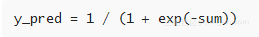y_pred即语音来自男性的概率。如果这个概率大于0.5，我们就认为这是男性的声音，否则，就认为是女性的声音。

# 在TensorFlow上实现分类器

在TensorFlow上使用该分类器，首先需要创建一个计算图（computational graph）。计算图由表示进行运算的节点（nodes）以及节点之间流动的数据（data）组成。逻辑斯蒂回归的图如下所示：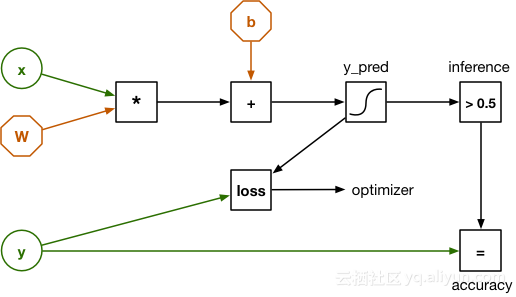这个图和前面的图看起来有一些区别，输入数据x不再是20个独立的数字，而是一个有20个元素的向量，权重用矩阵w表示，点积用简单的矩阵乘法代替。

由于权重初始化时全部置为0，分类器可能会做出错误的预测。所以，我们使用损失函数（loss function）来衡量分类器的分类水平。损失函数会比较预测结果y_pred和正确标签y。计算完训练样本的损失值（loss），我们使用反向传播（back propagation）修正权重w和b的值。训练过程要在所有样本上重复进行，直到计算图得出最佳权重数据。衡量分类器效果的损失值会随时间变得越来越小。

1.标量数字就是0阶张量；

2.向量是一阶张量；

3.矩阵是二阶张量；

4.三维数组是三阶张量

# 安装TensorFlow

环境：Python3.6

在Mac上使用Homebrew包管理器安装Python 3.6很简单，如果还没有安装Homebrew，可以在线安装。然后打开终端（Terminal）输入如下命令安装最新版Python：

brew install python3

然后使用Python的包管理器pip来安装所需要的包，在终端输入如下命令：

pip3 install numpy
pip3 install scipy
pip3 install scikit-learn
pip3 install pandas
pip3 install tensorflow

除了TensorFlow，我们还安装了Numpy, Scipy, pandas和scikit-learn库，这些包会安装在/usr/local/lib/python3.6/site-packages目录下，你可以随时查看。pip可以自动安装最适合你系统的TensorFlow版本。如果你想安装其它版本，请参照离线安装指南

下面测试一下所有的东西是否都已被正确安装。创建tryit.py，如下：

import tensorflow as tf

a = tf.constant([1, 2, 3])
b = tf.constant([4, 5, 6])

sess = tf.Session(config=tf.ConfigProto(log_device_placement=True))

print(sess.run(a + b))

然后从终端运行这个脚本，就会输出关于设备的一些调试信息，最可能是关于CPU的，如果你的Mac装有NVIDIA GPU，就会输出GPU相关的情况。最后会输出：

[5 7 9]


这是两个向量a和b的和。亦有可能出现如下信息：

W tensorflow/core/platform/cpu_feature_guard.cc:45] The TensorFlow library wasn't compiled to use SSE4.1 instructions, but these are available on your machine and could speed up CPU computations.

# 数据的详细分析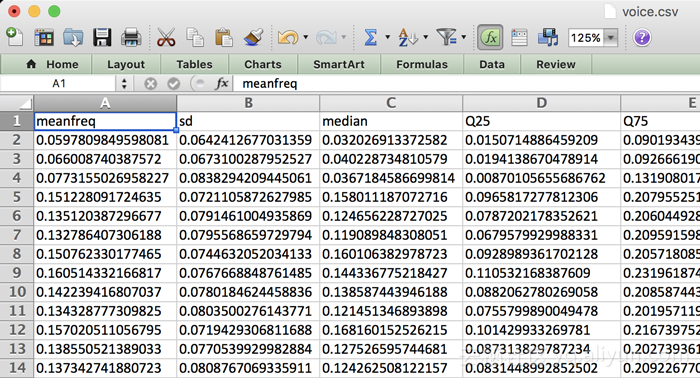Note: 这里需要指出深度学习和传统算法如逻辑斯蒂回归的区别。我们训练的分类器不能学习非常复杂的东西，需要在数据预处理阶段抽取特征。而深度学习系统可以直接将原始音频数据作为输入，抽取重要的声学特征，然后再进行分类。

# 创建训练集和测试集

        # This script loads the original dataset and splits it into a training set and test set.

import numpy as np
import pandas as pd

# Extract the labels into a numpy array. The original labels are text but we convert
# this to numbers: 1 = male, 0 = female.
labels = (df["label"] == "male").values * 1

# labels is a row vector but TensorFlow expects a column vector, so reshape it.
labels = labels.reshape(-1, 1)

# Remove the column with the labels.
del df["label"]

# OPTIONAL: Do additional preprocessing, such as scaling the features.
# for column in df.columns:
#     mean = df[column].mean()
#     std = df[column].std()
#     df[column] = (df[column] - mean) / std

# Convert the training data to a numpy array.
data = df.values
print("Full dataset size:", data.shape)

# Split into a random training set and a test set.
from sklearn.model_selection import train_test_split
X_train, X_test, y_train, y_test = train_test_split(data, labels, test_size=0.3, random_state=123456)

print("Training set size:", X_train.shape)
print("Test set size:", X_test.shape)

# Save the matrices using numpy's native format.
np.save("X_train.npy", X_train)
np.save("X_test.npy", X_test)
np.save("y_train.npy", y_train)
np.save("y_test.npy", y_test)


# 构建计算图

tf.name_scope（）将图的不同部分分成不同域，每个层都是在一个唯一的tf.name_scope（）下创建，作为在该作用域内创建的元素的前缀，x的独特名字将会是‘inputs/x-input’,这里将输入数据xy定义在inputs域下，分别命名为“x_input”和“y_put”，方便后面使用。

    with tf.name_scope("model"):
W = tf.Variable(tf.zeros([num_inputs, num_classes]), name="W")
b = tf.Variable(tf.zeros([num_classes]), name="b")

    y_pred = tf.sigmoid(tf.matmul(x, W) + b)

Note实际上，这行代码现在还没有计算任何东西，目前只是在构建计算图。这行代码将矩阵乘法和加法的节点，以及sigmod函数（tf.sigmoid）加入图中。当计算图构建完成时，创建一个TensorFlow会话（session），就可以测试真实数据了。

    with tf.name_scope("loss-function"):
loss = tf.losses.log_loss(labels=y, predictions=y_pred)
loss += regularization * tf.nn.l2_loss(W)

log_loss节点接收样本数据的真实标签y作为输入，与预测值y_pred比较，比较的结果代表损失值（loss）。第一次训练时，在所有的样本上预测值y_pred都会是0.5，因为分类器现在并不知道真实答案。初始损失值为-ln(0.5)，即0.693146,。随着不断训练，损失值会变得越来越小。

    with tf.name_scope("hyperparameters"):
regularization = tf.placeholder(tf.float32, name="regularization")
learning_rate = tf.placeholder(tf.float32, name="learning-rate") 

    with tf.name_scope("train"):
train_op = optimizer.minimize(loss)

    with tf.name_scope("score"):
correct_prediction = tf.equal(tf.to_float(y_pred > 0.5), y)
accuracy = tf.reduce_mean(tf.to_float(correct_prediction), name="accuracy")

    with tf.name_scope("inference"):
inference = tf.to_float(y_pred > 0.5, name="inference")



# 训练分类器

with tf.Session() as sess:
tf.train.write_graph(sess.graph_def, checkpoint_dir, "graph.pb", False)

sess.run(init)

step = 0
while True:
# here comes the training code

while True:循环内，操作如下：

        perm = np.arange(len(X_train))
np.random.shuffle(perm)
X_train = X_train[perm]
y_train = y_train[perm]

        feed = {x: X_train, y: y_train, learning_rate: 1e-2,
regularization: 1e-5}
sess.run(train_op, feed_dict=feed)

train_op节点会运行很多次，反向传播机制每次都会对权重w和偏置b进行微调，随着迭代次数增多，wb就会逐渐达到最优值。为了帮助理解训练过程，在每迭代1000次时，运行accuracyloss节点，输出相关信息：

if step % print_every == 0:
train_accuracy, loss_value = sess.run([accuracy, loss],
feed_dict=feed)
print("step: %4d, loss: %.4f, training accuracy: %.4f" % \
(step, loss_value, train_accuracy))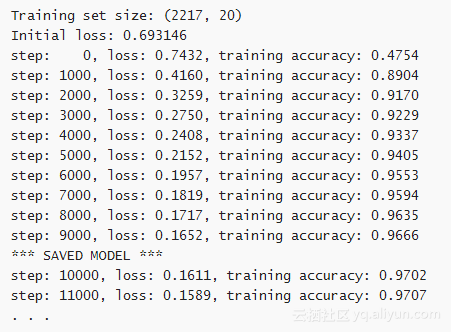# 分类器效果

Note: 测试集上的准确率会比训练集中的准确率（97%）低，但是不应该太低。如果你的训练器出现过拟合，那就需要重新调整训练过程了。

还是先导入包，然后载入测试数据：

import numpy as np
import tensorflow as tf
from sklearn import metrics

y_test = np.load("y_test.npy")

  with tf.Session() as sess:
graph_file = os.path.join(checkpoint_dir, "graph.pb")
with tf.gfile.FastGFile(graph_file, "rb") as f:
graph_def = tf.GraphDef()
tf.import_graph_def(graph_def, name="")

TensorFlow推荐使用*.Pb保存数据，所以这里只需要一些辅助代码就可以载入这个文件，并导入会话（session）中。再从检查点文件中载入wb的值：

W = sess.graph.get_tensor_by_name("model/W:0")
b = sess.graph.get_tensor_by_name("model/b:0")

checkpoint_file = os.path.join(checkpoint_dir, "model")
saver = tf.train.Saver([W, b])
saver.restore(sess, checkpoint_file)

    x = sess.graph.get_tensor_by_name("inputs/x-input:0")
y = sess.graph.get_tensor_by_name("inputs/y-input:0")
accuracy = sess.graph.get_tensor_by_name("score/accuracy:0")
inference = sess.graph.get_tensor_by_name("inference/inference:0")

    feed = {x: X_test, y: y_test}
print("Test set accuracy:", sess.run(accuracy, feed_dict=feed))

predictions = sess.run(inference, feed_dict={x: X_test})
print("Classification report:")
print(metrics.classification_report(y_test.ravel(), predictions))
print("Confusion matrix:")
print(metrics.confusion_matrix(y_test.ravel(), predictions))
在终端运行test.py，结果如下：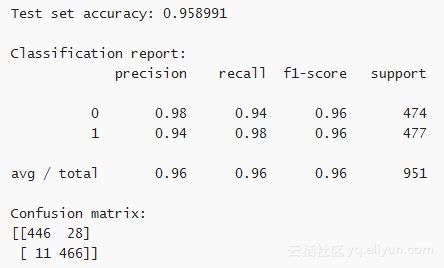ios开发学习--按钮（Button）效果源码分享--系列教程2
903 0http://code4app.com/
737 0centos6.9 编译openJdk源码

896 0pinyin4j项目  官网地址 http://pinyin4j.sourceforge.net/   我们先把资源下载下来，连同源码和jar包一起放入工程。如下图：   接下来在demo包下，我们写一个测试类，简单使用pinyin4j对中文字符进行自然排序 新建一个ConvertTest.
1066 0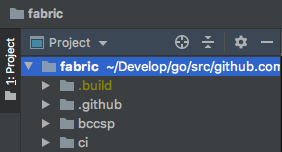hyperledger fabric 源码调试(orderer)环境搭建教程

1606 0《TensorFlow技术解析与实战》——2.4　从源代码安装
1500 0Microsoft Visual Studio与Firefly 一直提示加载项目，更新源码状态问题
笔记本一开始安装的是vs2010，由于近期开发要用vs2008与vs2005于是今天又把2008、2005安装上了，但在打开项目的时候，先是提示加载项目文件，然后一直提示更新源码状态，很慢很慢的，之前只有vs2010的时候，打开是很快的，现在不管是用2008、2005、2010就没有一个快的，源码管理用的是firefly，有人知道为什么会出现这种情况吗？
991 0ios开发学习--动画（Animation）效果源码分享--系列教程1
1149 0627 0Tensorflow源码解析4 -- 图的节点 - Operation
# 1 概述 上文讲述了TensorFlow的核心对象，计算图Graph。Graph包含两大成员，节点和边。节点即为计算算子Operation，边则为计算数据Tensor。由起始节点Source出发，按照Graph的拓扑顺序，依次执行节点的计算，即可完成整图的计算，最后结束于终止节点Sink，并输出计算结果。 本文会对节点Operation进行详细讲解。 # 2 前端节点数据
728 0
【方向】

696

5

《SaaS模式云原生数据仓库应用场景实践》

《看见新力量：二》电子书